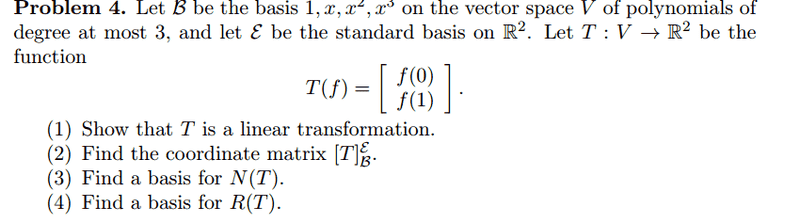# Finding N(T) and R(T)

Alright this problem has really gotten me confused. I skipped 1 and 2 because I know how to do those, but 3 and 4, I do not.

I think the problem statement is saying the linear transformation transforms the vector space V to R^2. and it's defined by T(f)=....

For 3) find a basis for N(T)

The book defines N(T) as = {v ε V l T(v)=0}
So I must find T such that T(v)=0.

I have no clue how to do this problem. can someone give me a hint?
I've looked at other types of problems where we find a basis for N(T) and those seem easy, but this one just got me confused.

What is f?

SammyS
Staff Emeritus
Homework Helper
Gold Member
Alright this problem has really gotten me confused. I skipped 1 and 2 because I know how to do those, but 3 and 4, I do not.

I think the problem statement is saying the linear transformation transforms the vector space V to R^2. and it's defined by T(f)=....

For 3) find a basis for N(T)

The book defines N(T) as = {v ε V l T(v)=0}
So I must find T such that T(v)=0.

I have no clue how to do this problem. can someone give me a hint?
I've looked at other types of problems where we find a basis for N(T) and those seem easy, but this one just got me confused.

What is f?
Where are the problems?

I see none !